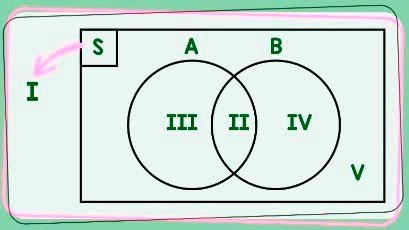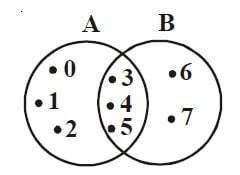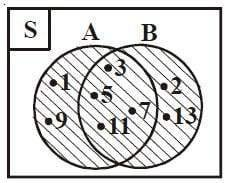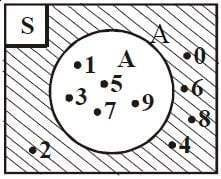# What is Venn Diagram ? (Complete Explanation and Examples of Use)

A Venn diagram is an image used to express the relationship between sets in a group of objects that have something in common.

Typically, Venn diagrams are used to describe sets that intersect, are independent of each other and so on. This type of diagram is used for scientific and technical data presentation which is useful in the fields of mathematics, statistics and computer applications.

In order to understand the Venn diagram, there is a set in it that must be understood first.

## The Set

A set is a clearly defined collection of objects.

For example, the clothes you are wearing today are a set, which includes hats, shirts, jackets, pants and so on

You can write a set with parentheses, as follows

{hats, clothes, jackets, pants,…}

You can also write sets in numbers like

• The set of all numbers: {0,1,2,3…}
• Set of prime numbers: {2,3,5,7,11,13,…}

Simple isn’t it?

The Venn diagram which contains the set is depicted in diagrammatic form so that it is easy to understand. How to draw a diagram as shown below.## How to draw a Venn diagram

1. The universal set in the Venn diagram is represented as a rectangular shape.
2. Each set being described is described as a closed circle or curve.
3. Each member of the set is represented in dots or dots.

The venn diagram has several shapes, for more details, see the following explanation,

## Venn Diagram Shape

1. The sets intersect each other

This venn diagram is depicted where two sets intersect each other because they have similarities. For example, if there is a set A and B, both of them intersect each other if they have the same thing, this means that members who enter set A are also included in set B.

Set A intersects set B can be written A∩B.

2. The sets are mutually exclusive

Sets A and B can be said to be independent of each other if the members of set A are not the same as members of set B. This independent set can be written as A // B.

3. Subsets

Set A can be said to be part of set B if all members of set A are members of set B.

4. The set of the same

This venn diagram states that if sets A and B consist of the same set members, then we can conclude that each member B is a member of A. Example A = {2,3,4} and B = {4,3,2} are the same set then we can write it A = B.

5. Equivalent sets

Sets A and B are said to be equivalent if the number of members of the two sets is the same. Set A is equivalent to set B which can be written n (A) = n (B).

## Diagram Venn Relationships

In a venn diagram, there are four relationships between sets including slices, combinations, set complement and set differences.

### Intersections

The intersection sets A and B (A∩B) are sets whose members are in set A and set B.For example, set A = {0,1,2,3,4,5} and set B = {3,4,5,6,7}. note that in both sets there are two common members, namely 3,4 and 5. Now, from this similarity it can be said that the slices of sets A and B are written as (A∩B) = {3,4,5}.

### Union

A union of sets A and B (written as A ∪ B) is a set whose members are set A or members of set B or members of both. The Union of sets A and B is denoted by A ∪ B = {x | x ∈ A or x ∈ B}For example the sets A = {1,3,5,7,9,11} and B = {2,3,5,7,11,13}. If set A and set B are combined, a new set will be formed whose members can be written as A ∪ B = {1,2,3,5,7,9,11,13}.

### Complement

The complement of set A (written as Ac) is a set whose members are members of the set universe but not members of set A.For example S = {0, 1, 2, 3, 4, 5, 6, 7, 8, 9} and A = {1, 3, 5, 7, 9}. We can note that all members of S which are not members of A form a new set, namely {0,2,4,6,8}. Then the complement of set A is Ac = {0,2,4,6,8}.